Reconstruction of the 2014 Tetbury Lane formation 1.Draw a circle. Draw and extend the horizontal and vertical centerlines. 2.Draw the righthand ray of circle 1, from its center to its righthand intersection with the horizontal centerline. Divide this line into five equal parts. 3.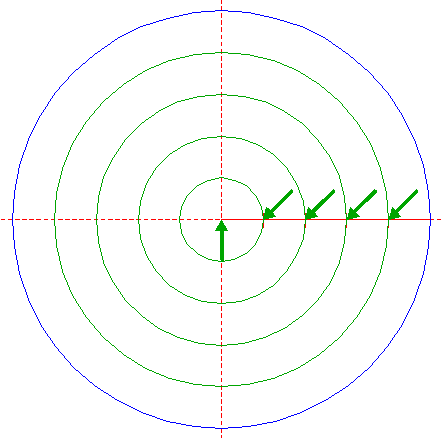Construct four circles concentric to circle 1, passing through division nodes 2. 4.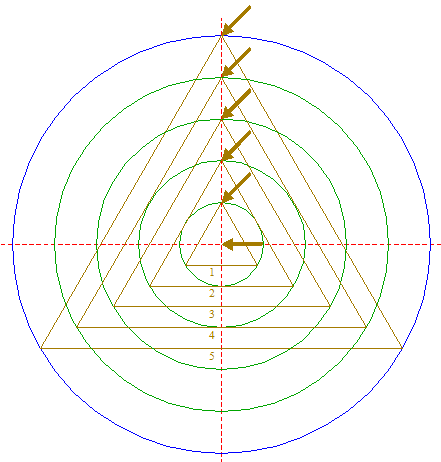Construct the five inscribed equilateral triangles of circles 1 and 3, all pointing up. Number the triangles 1 - 5 from inside outwards. 5.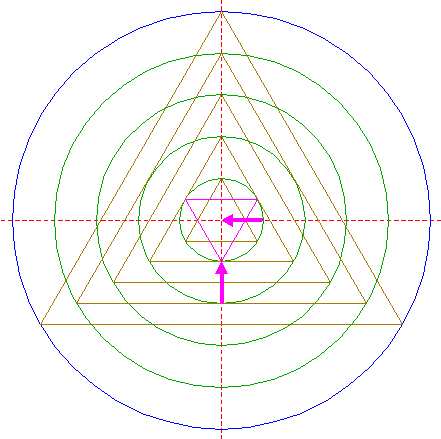Construct the inscribed equilateral triangle of innermost circle 3, pointing down. 6.Draw the connecting line between the righthand angular points of triangle 4 nr. 1 and triangle 5. 7.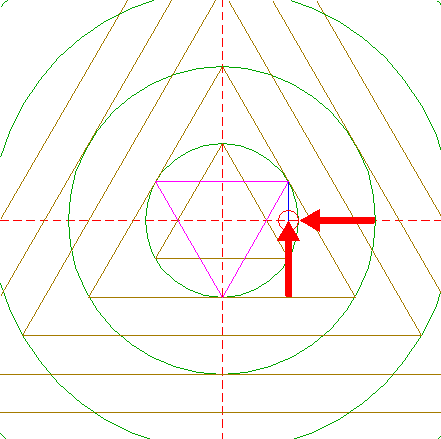Construct a circle centered at the intersection of line 6 and the horizontal centerline, tangent to innermost circle 3 at the righthand side. 8.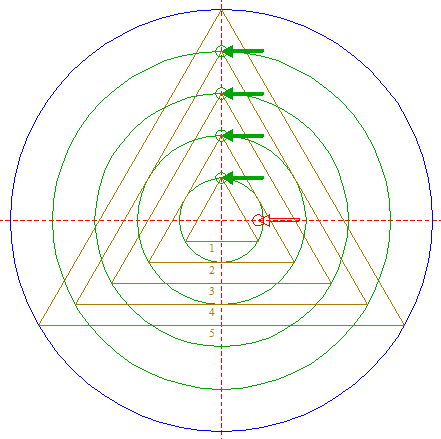Copy circle 7 four times, to the tops of triangles 4 nrs. 1 - 4. 9.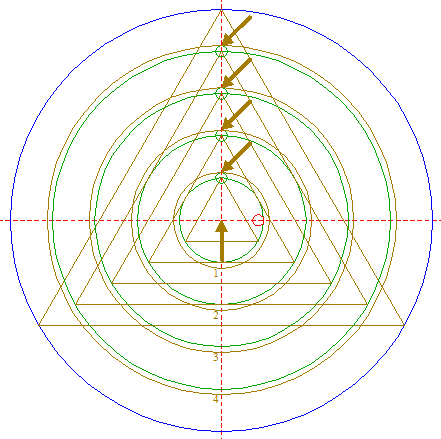Construct four circles concentric to circle 1, tangent to circles 8 at the upper sides. Number these circles 1 - 4 from inside outwards. 10.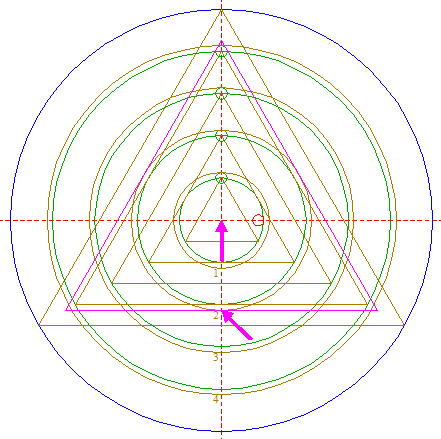Construct the circumscribed equilateral triangle of circle 9 nr. 2, pointing up. 11.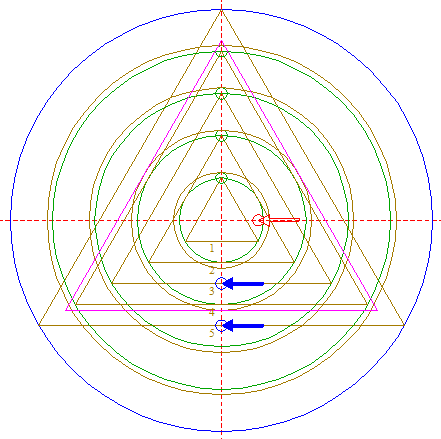Copy circle 7 two times, to the mid-points of the lower sides of triangles 4 nrs. 3 and 5. 12.Construct two circles concentric to circle 1, tangent to circles 11 at the lower sides. 13.Construct the two circumscribed equilateral triangles of circles 12, pointing up. 14.Construct a "two-points" circle (defined by the two end-points of a centerline) between the center of circle 7 and its righthand intersection with the horizontal centerline. 15.Construct a "two-points" circle between the center of circle 14 and its righthand intersection with the horizontal centerline. 16.Copy circle 15 four times, to the center of circle 1, and to the angular points of outermost triangle 13. 17.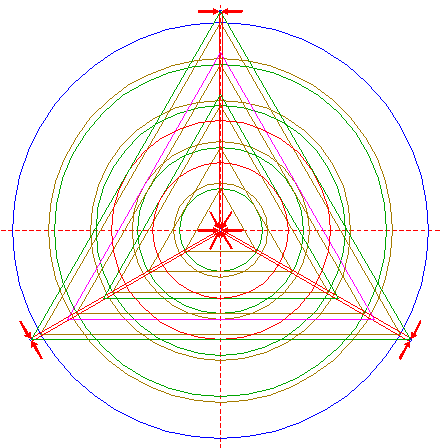Construct three pairs of parallel lines, tangent to central circle 16, and two by two tangent to the other circles 16, all at both sides, as shown. See detail.18.Copy circle 7 to the top of outermost triangle 13. See detail.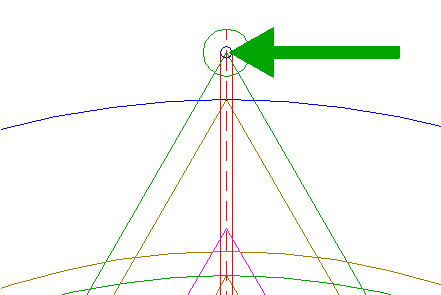19.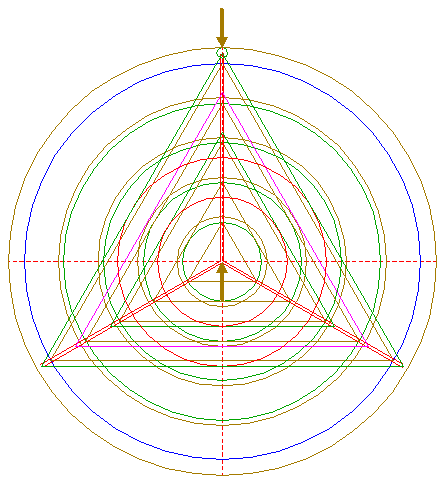Construct a circle concentric to circle 1, tangent to circle 18 at the upper side. 20.Circles 3, 9 and 19, triangles 4, 10 and 13, and lines 17, are used for the final reconstruction. 21.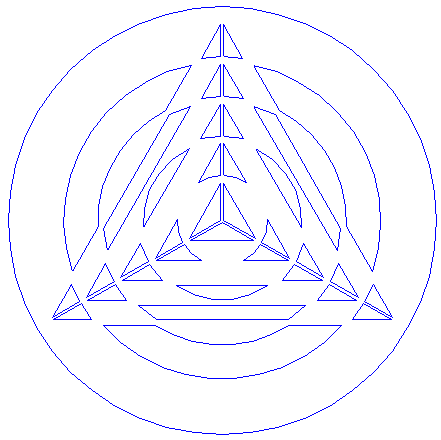Remove all parts not visible within the formation itself. 22.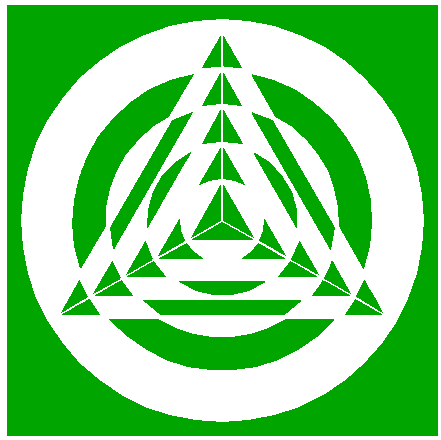Colour all areas corresponding to standing... 23....or to flattened crop, and finish the reconstruction of the 2014 Tetbury Lane formation. 24.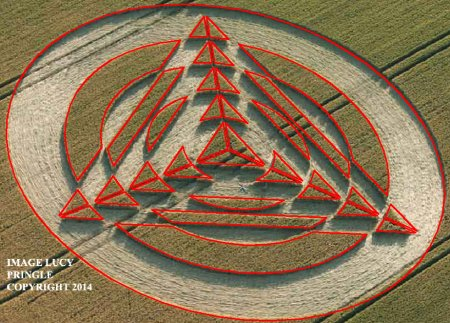The final result, matched with the aerial image.
 Copyright © 2014, Zef Damen, The Netherlands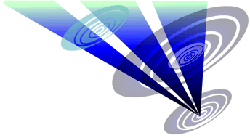BACKMark Fussell & Stuart Dike